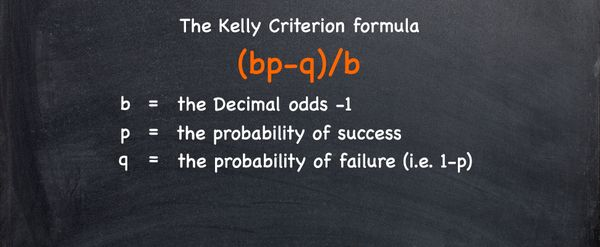# How to use Kelly Criterion for betting

#### Bettors should always look for a mathematical edge rather than rely on their impulses. Learning how to use the Kelly Criterion, for example, is a great way for bettors to determine how much they should stake. Read on to find out.Prior to placing a bet bettors should consider six important questions: who, what, when, where, why and how? But for this article, it is the how, as in how much to bet, we are interested in.

Consider placing a bet on the English Premier League. We can adapt these questions accordingly:

•        Who to bet on? Manchester United
•        What to bet on? Top 4 finish
•        When to bet on? Now
•        Where to bet on? Pinnacle Sports tend to offer the best odds
•        Why to bet on? They seem to be under-priced.
•        How … how much to bet on this outcome?

Most articles focus on the first five questions, typically using mathematical or statistical justifications on answering ‘why’ – such as the article on how to use Monte Carlo methods.

In making financial decisions, the key issue is not only finding the adequate financial products to invest in but also deciding how to subdivide one’s portfolio. Similarly, an important question for a bettor, is how much to wager?

Many papers recommend using the Kelly Criterion or a derivative of it – such as my 2013 papaer appearing in the The Journal of Gambling Business and Economics. In essence, the Kelly Criterion calculates the proportion of your own funds to bet on an outcome whose odds are higher than expected, so that your own funds grow exponentially.

#### The Kelly Criterion formula is: (bp-q)/b

B = the Decimal odds -1
P = the probability of success
Q = the probability of failure (i.e. 1-p)

### Using a coin as an example of Kelly Criterion staking

For example, consider you are betting on a coin to land on heads at 2.00. However, the coin is biased and has a 52% chance of ending up on heads.

In this case:

P= 0.52
Q = 1-0.52 = 0.48
B = 2-1 = 1.

This works out at: (0.52×1 – 0.48) / 1 = 0.04

Therefore the Kelly Criterion would recommend you bet 4%. A positive percentage implies an edge in favour of your bankroll, so your funds grow exponentially. You can also test the criterion for different values in this online sheet by using the code below.

Decimal Odds:
Probability of Success:
Percentage of own stake: 0
A negative percentage implies that you should not wager on this outcome.

Ultimately the Kelly criterion offers a distinct advantage over other staking methods such as Fibonacci and Arbitrage methods as there is a lower risk. However, it does require precise calculation of the likelihood of an event outcome, and discipline of this method will not provide explosive growth of your bankroll.

### MORE: TOP 20 Bookmakers that accept Cryptocurrency >>>

Source: pinnacle.com